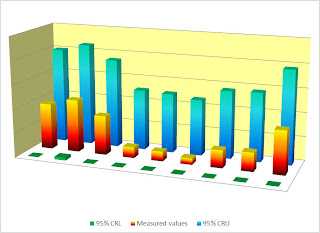# Born krigers do more with less

Teaching statistics to born krigers takes a long time. What krigers do best is fit curves. Think what happens when a curve is fitted through a set of measured values. Most of all there is much pride and joy. A perfect curve is indeed a thing of beauty. Look at the Fourier transform of Wölfer annual sunspot counts from 1700 to 1987. Isn’t it as stunning as the original plot? It does put into perspective the power of mathematics when applied to an ordered set of measured values in our own sample space of time. A perfect fit is of less interest in my work than the statistics behind ordered sets of measured values. For example, I applied mathematical statistics to derive the statistics of Wölfer annual sunspot counts for the period from 1749 to 1924. I work with spreadsheet software because it is such a powerful tool to show and tell. Several Excel files are posted on my website under Statistics for geoscientists.

Wiki’s Kriging doesn’t test for spatial dependence between measured values in an ordered set. Wiki’s keepers of Krige’s grail didn’t even try. Here’s what they wrote about kriging, “The theory behind interpolation and extrapolation by Kriging was developed by the French mathematician Georges Matheron based on the Master’s thesis of Daniel Gerhardus Krige.” It’s short and crisp but not to the point.

Krige’s 1951 Master thesis brings up ‘knowledge of mathematical statistics’, ‘careful statistical analysis’, ‘science of statistics’, ‘modern statistical basis’, ‘application of statistics’, and so on. It does read like a thesis on statistics, doesn’t it? Nowhere did Krige bring up ‘geostatistics’. A 2003 Tribute to Krige alluded to “…his pioneering work in the application of mathematical statistics…” The same tribute alluded to Krige’s 1952 paper in which he “introduced, inter alias, the basic geostatistical concepts of ‘support’, ‘spatial structure’, ‘selective mining units’, and ‘grade-tonnage curves’. Did it take Krige one year and a bit of inter alias to switch from real statistics to a pinch of between-the-lines geostatistics? Not quite! He was a committed geostatistician when he wrote the Preface to David 1977 Geostatistical Ore Reserve Estimation. But when did Krige really take to kriging?

Matheron’s Note Statistique No 1 saw the light of day in North Africa on November 25, 1954. He coined the first krige-inspired eponym in his 1960 Krigeage d’un panneau rectangulaire par sa périphérie. Matheron didn’t refer to Krige’s 1951 Master thesis. Neither did he much refer to anyone’s work but his own. In those early days Matheron himself dawdled between statistics and geostatistics. But he was not much of a statistician even though he thought he was one.

It makes sense to compare Wiki’s Kriging with Krige’s teachings. Look at tFigure 1 in Wiki’s Kriging. The graph didn’t irk me quite as much as did the confidence intervals for measured values. Once upon a time I tried to get the set of measured values that underpin Figure 1 but its caretaker(s?) didn’t respond. So, I waited until it was time to take a stand against junk statistics on Wikipedia.Figure 1

Example of one-dimensional data interpolation by kriging, with confidence intervals.
Squares indicate the location of the data.
The kriging interpolation is in red.
The confidence intervals are in green.

I enlarged Figure 1 and measured X- and Y-coordinates for all points in mm. I tested for spatial dependence by applying Fisher’s F-test to the variance of the set and the first variance term for the ordered set. I applied weighting factors because of unevenly spaced measured values. That’s why degrees of freedom become irrational numbers.Given that the observed value of F=var1(x)/var(x)=1,504/1,408=1.07 is below the tabulated value of F0.05;dfo;df=6.04, it follows that the ordered set of measured values does not display a significant degree of spatial dependence. Hence, measured values in the ordered set are randomly distributed within this sample space. Therefore, interpolation between measured values makes as much sense as extrapolation beyond the set. As a matter of fact, it does give junk statistics of the worst kind wherever and whenever randomness rules. I do not know how Wiki’s Kriging caretakers cooked up the confidence intervals in Figure 1. Next, I applied plain vanilla statistics and plotted confidence intervals in this bar chart.The bars in this graph, unlike the measured values in Figure 1, are evenly spaced. I’ll show in another block that interpolation between measured values in an ordered set does indeed give the same sort of junk statistics as did Bre-X and the kriging game.